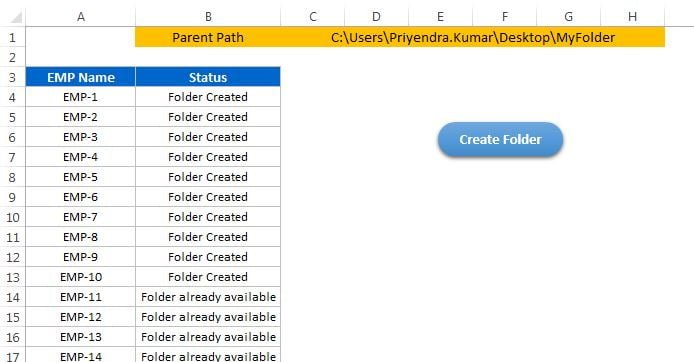# Create Multiple Folders from Excel Range

In the previous article you have learnt how to check the whether a folder exist or not. In this article you will learn we can create multiple folders on one click using excel range.### Below the VBA code to create multiple folder from excel sheet

```Option Explicit

Sub Create_Multiple_Folder()

Dim sh As Worksheet

Set sh = ThisWorkbook.Sheets("Sheet1")

Dim sub_folder_path As String

Dim i As Integer

For i = 4 To sh.Range("A" & Application.Rows.Count).End(xlUp).Row

sub_folder_path = sh.Range("C1").Value & Application.PathSeparator & sh.Range("A" & i).Value

If Dir(sub_folder_path, vbDirectory) = "" Then

MkDir (sub_folder_path)

sh.Range("B" & i).Value = "Folder Created"

Else

sh.Range("B" & i).Value = "Folder already available"

End If

Next i

End Sub```